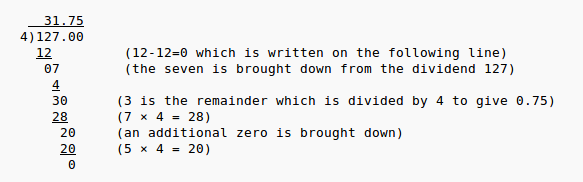Check out our latest project: Algebrarules.com. The Rules of Algebra, Sweet 'n Simple. X

Sort By: Formula per Page:

By PiThagoras

Tags: Long Division, Division, Quotient, Remainder, Divisor,...

1

Posted: 2013-04-09
Long Division Procedure
Long Division Procedure1. When dividing two numbers, for example, n divided by m, n is the dividend and m is the divisor; the answer is the quotient.
2. Find the location of all decimal points in the dividend and divisor.
3. If necessary, simplify the long division problem by moving the decimals of the divisor and dividend by the same number of decimal places, to the right, (or to the left) so that the decimal of the divisor is to the right of the last digit.
4. When doing long division, keep the numbers lined up straight from top to bottom under the tableau.
5. After each step, be sure the remainder for that step is less than the divisor. If it is not, there are three possible problems: the multiplication is wrong, the subtraction is wrong, or a greater quotient is needed.
6. In the end, the remainder, r, is added to the growing quotient as a fraction, $r/m$